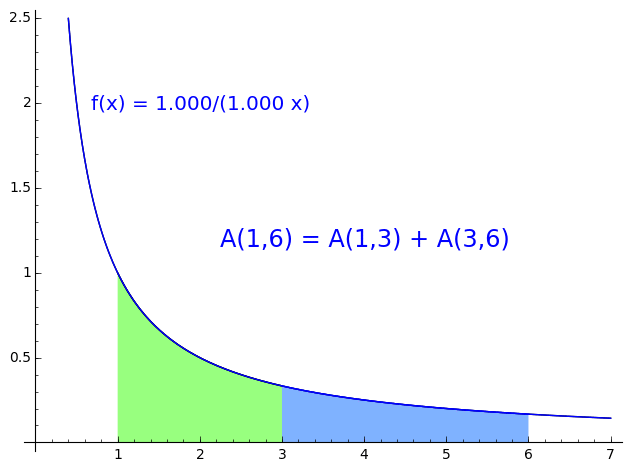# Fun Topics in Elementary Calculus

The ideas of calculus appear in elemenary mathematics. On this page, I collect some of the ones I've thought about. Enjoy!

## Elementary introduction to the logarithm rule¶

The logarithm rule $\log(st) = \log(s) + \log(t)$ comes from the geometry of the hyperbola $$xy = 1$$. Let $$A(a,b)$$ denote the area between $$a$$ and $$b$$ under the curve $$y = 1/x$$ and consider the animation here.

In the first half, the blue area is scaled horizontally by a factor of $$1/3$$ and $$1/x$$ changes to $$1/(3x)$$. Then the blue area is scaled vertically by a factor of $$3$$ and $$1/(3x)$$ changes to $$3/(3x)$$, which is the same as $$1/x$$. The total scaling of the blue area area is the product of the two factors, $$3 * 1/3 = 1$$ ! (excitement)The area under $$1/x$$ satisfies the logarithm rule.

tags: fun-topics